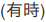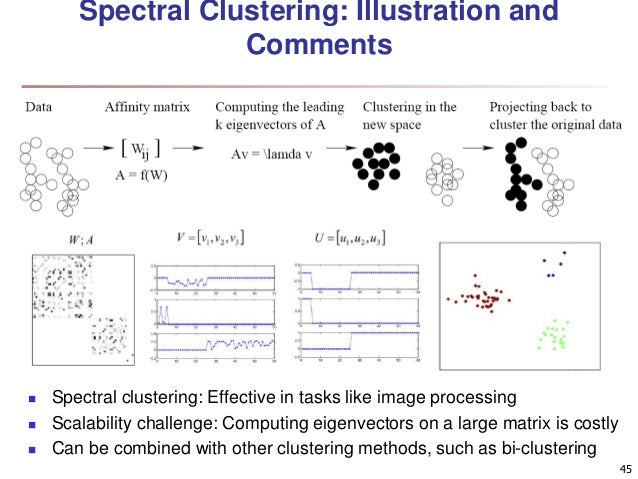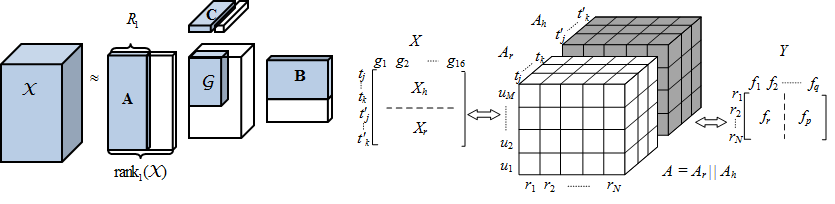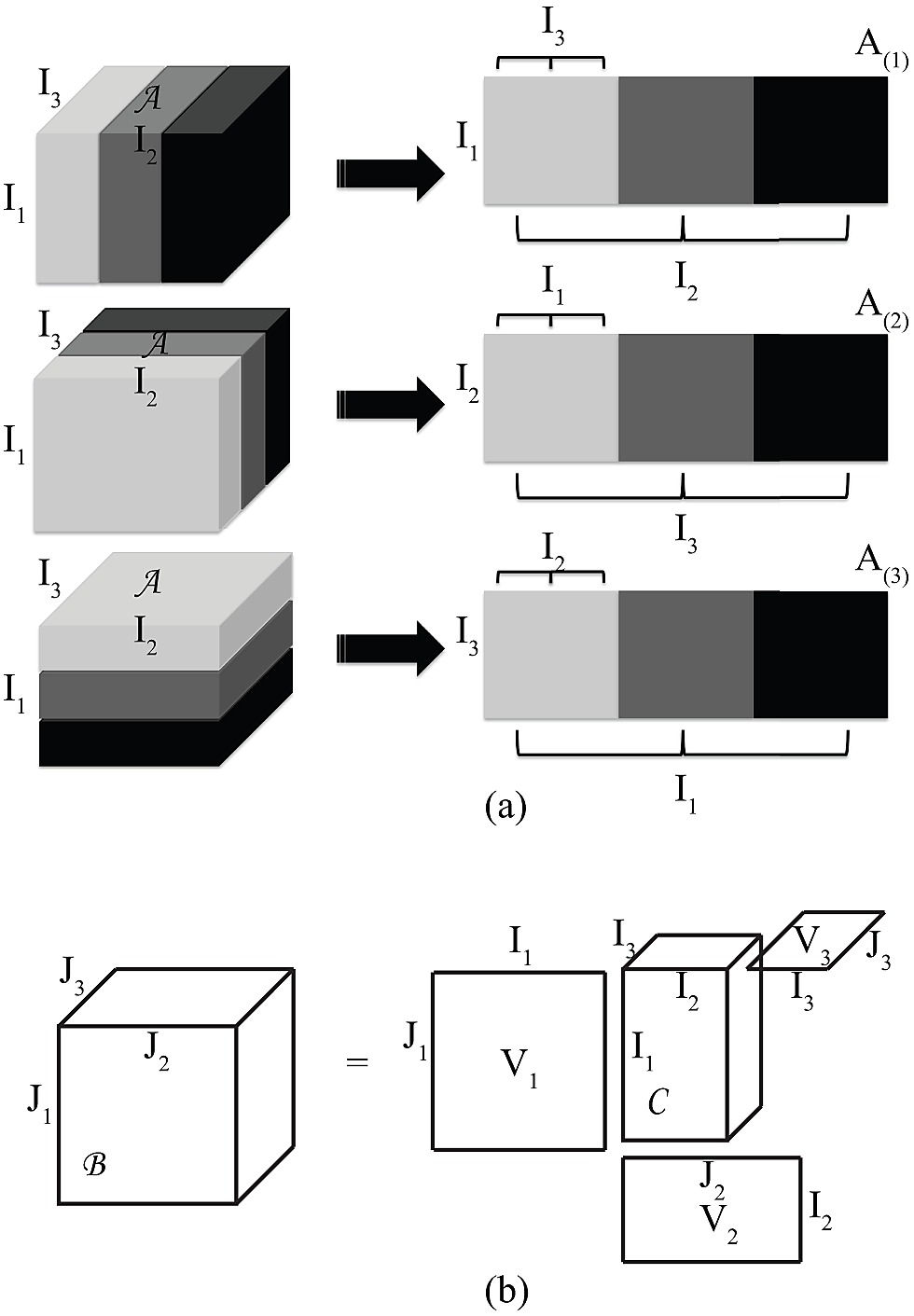// Databricks notebook source exported at Tue, 28 Jun 2016 10:36:27 UTC

# Scalable Data Science

## Course Project - High Order Spectral Clustering

### by Xin Zhao

The html source url of this databricks notebook and its recorded Uji:### 1. Spectral clustering

#### 1.1 What is spectral clustering

In multivariate statistics and the clustering of data, spectral clustering techniques make use of the spectrum (eigenvalues) of the similarity matrix of the data to perform dimensionality reduction before clustering in fewer dimensions.

The similarity matrix is provided as an input and consists of a quantitative assessment of the relative similarity of each pair of points in the dataset. Such measure is used to transform data to overcome difficulties related to lack of convexity in the shape of the data distribution. The measure gives rise to an (n, n)-sized similarity matrix for a set of (n,d) points, where the entry (i,j) in the matrix can be simply the Euclidean distance between i and j, or it can be a more complex measure of distance such as the Gaussian. Further modifying this result with network analysis techniques is also common.

In mathematics, spectral graph theory is the study of properties of a graph in relationship to the characteristic polynomial, eigenvalues, and eigenvectors of matrices associated to the graph, such as its adjacency matrix or Laplacian matrix.#### 1.2 Spectral clustering with different clustering method

The following two examples show two different Spectral clustering method as they uses different clustering algorithm:#### 1.3 Compare variaty clustering methods### 2. High Order Spectral Clustering

#### 2.1 Why high order

• kernel principal component analysis (kernel PCA) in not enough in multivariate analysis
• linkage to the original feature

#### 2.2 How

• high order spectral cluster diagrams
• Data –> Graph/Similarity Tensor –> Compute leading K (vector) svd and core tensor –> Clustering on the new latent tensor –> Project clusters back to original data

#### 2.3 Tensor

• What is tensor• High order SVD• Tensor unfoldingPlease refer to De Lathauwer, L., De Moor, B. and Vandewalle, J. (2000) for details in high order singular decomposition.

• The size of (n,d) increase to (n,n,d), which means very expensive in memory and computation performance. E.g, (100k,100k) double requires about 80G
• Not suitble for large number of clusters
• Difficult to do out of sample embedding.

### High Order Spectral Clustering Package

In this project, a high order spectral clustering package is developed as attached spectralclustering_01.jar. The package is implemented as:

• Implement in Scala with Apache Spark
• Tensor implemented as both distributed and non-distributed frame
• The clustering method is K-means
• The current implementation can handle continuous and discrete features but not catergorical features

#### Sample code

• The following is just some sample source code of the library “high order spectral clustering”
``````package tensorSVD

import org.apache.spark.SparkContext
import org.apache.spark.mllib.linalg.distributed.IndexedRowMatrix
import org.apache.spark.rdd.RDD

/**
* Define RDD Tensor Class
* @constructor create rdd tensor class which contains dimension, values in Rdd and others
* @param dim1 first dimension
* @param dim2 second dimension
* @param dim3 third dimension, keep the smallest dimension as the third
*/
class RddTensor(dim1: Int, dim2: Int, dim3: Int){
//tensor vale is defined as a RDD of Tuple3(dim3Index: Int, rowIndex: Int, rowValue: Array[Double])
//Note: Keep dim3 as the smallest dimension
//      Keep dim1 as the most important dimension such as along sample point

//Second constructor
def this(dims: Array[Int])=this(dims(0),dims(1),dims(2))

val dim=Array(dim1,dim2,dim3)
//class field
//val dim=(dim1,dim2,dim3) //dimension of tensor
var nonemptyDim3 = 0
var values: RDD[(Int,Int,Array[Double])]=null
var isSymmetric=true //if every matrix along dim3 A(:,:,i) is symetrix

/**
* The method add value into the rdd Tensor. The input must be dim1*dim2 values (as RddMatrix)
* @param newRddmat the new value matrix to be added in
* @param dim3Index the third dimension index
*/

def addDim3(newRddmat: RddMatrix, dim3Index: Int) {
if(dim3Index>=this.dim(2)){
throw new IllegalArgumentException("arg 2 need be in range of 0 to dim3 of the tensor class")
}
if(newRddmat.cols!=this.dim(1) || newRddmat.rows!=this.dim(0)){
throw new IllegalArgumentException("the adding matrix dimension is not match the tensor")
}
if(this.nonemptyDim3==0){
this.values=newRddmat.values.map(v=>(dim3Index,v._1,v._2))
}else{
this.values=this.values.union(newRddmat.values.map(v=>(dim3Index,v._1,v._2)))
}
this.nonemptyDim3 +=1
}

/**
* The method unfold the rdd tensor along the required order (0,1 or 2)
* @param dimi the unfold direction/order (0,1 or 2)
* @param sc the spark context
* @return the result matrix (RddMatrix)
*/
def unfoldTensor(dimi: Int, sc: SparkContext): RddMatrix = {
val dims=this.dim
var outmat:RddMatrix = null

if(dimi==0){
val rmat:RddMatrix = new RddMatrix(this.dim(dimi),0)
rmat.setValues(this.values.map(v=>(v._2,(v._1,v._3))).groupByKey()
.map(x=>(x._1,x._2.toArray.sortBy(_._1))).map(b=>(b._1,b._2.flatMap(bi=>bi._2))))
outmat=rmat
}
if(dimi==1){
val rmat:RddMatrix = new RddMatrix(this.dim(dimi),0)
if(this.isSymmetric){
val dd=2
val temp1=Range(0,2).toArray
val temp2=Range(0,3).toArray
val indx: IndexedSeq[Int]=temp1.map(v=>(v,temp2)).flatMap(v=>v._2.map(_*dd+v._1))
rmat.setValues(this.values.map(v=>(v._2,(v._1,v._3))).groupByKey()
.map(x=>(x._1,x._2.toArray.sortBy(_._1)))
.map(b=>(b._1,b._2.flatMap(bi=>bi._2.zipWithIndex)))
.map(v=>(v._1,v._2.sortBy(_._2).map(vi=>vi._1))))
outmat=rmat
}else{
throw new Exception("Folding for dim2 not apply to asymmetric tensor in dim1 by dim2")
}
}
if(dimi==2){
val rmat:RddMatrix = new RddMatrix(this.dim(0)*this.dim(1),this.dim(dimi))
//Note: as dim(2) is small, this returns the transpose of the unfold matrix
val cc=this.dim(1)
rmat.setValues(this.values.flatMap(v=>v._3.zipWithIndex.map(vi=>(v._1,v._2,vi._2,vi._1)))
.map(b=>((b._2,b._3),(b._1,b._4))).groupByKey()
.map(x=>(x._1._1*cc+x._1._2,x._2.toArray.sortBy(_._1).map(b=>b._2))))
outmat=rmat

}
outmat
}

/**
* The method fold a matrix back to rdd tensor for along the required order/dim
* @param rddMat the input rdd matrix to be folded
* @param dimi the folding order
* @return the folded rdd tensor
*/
def foldTensorDimOne(rddMat:IndexedRowMatrix, dimi:Int) :RddTensor = {
if(dimi!=1){
throw new IllegalArgumentException("The fold method of rddTensor only available along the first dimension")
}
val ndim=this.dim.length
val size=this.dim
//val tempMat=rddMat.rows.map
val result: RddTensor = null
result
}

}

...

``````

Updated: